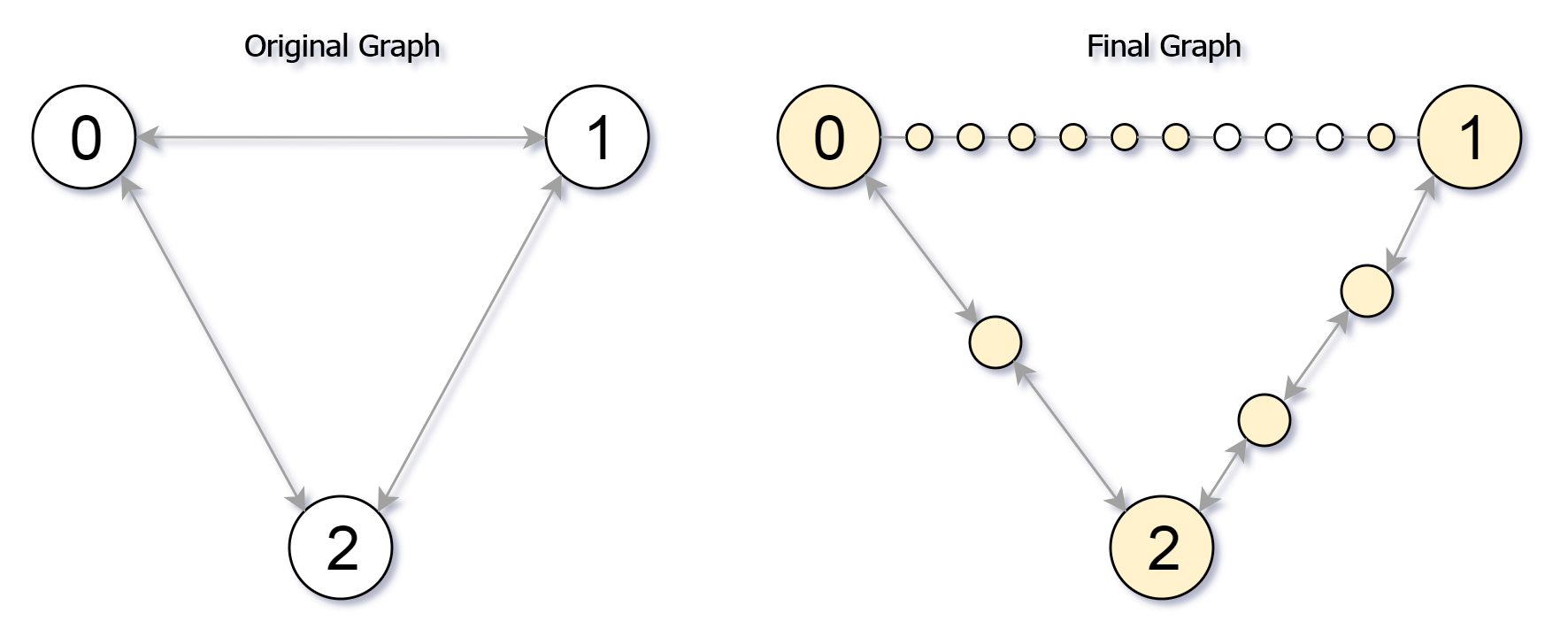## Algorithm

Problem Name: 882. Reachable Nodes In Subdivided Graph

You are given an undirected graph (the "original graph") with `n` nodes labeled from `0` to `n - 1`. You decide to subdivide each edge in the graph into a chain of nodes, with the number of new nodes varying between each edge.

The graph is given as a 2D array of `edges` where `edges[i] = [ui, vi, cnti]` indicates that there is an edge between nodes `ui` and `vi` in the original graph, and `cnti` is the total number of new nodes that you will subdivide the edge into. Note that `cnti == 0` means you will not subdivide the edge.

To subdivide the edge `[ui, vi]`, replace it with `(cnti + 1)` new edges and `cnti` new nodes. The new nodes are `x1`, `x2`, ..., `xcnti`, and the new edges are `[ui, x1]`, `[x1, x2]`, `[x2, x3]`, ..., `[xcnti-1, xcnti]`, `[xcnti, vi]`.

In this new graph, you want to know how many nodes are reachable from the node `0`, where a node is reachable if the distance is `maxMoves` or less.

Given the original graph and `maxMoves`, return the number of nodes that are reachable from node `0` in the new graph.

Example 1:```Input: edges = [[0,1,10],[0,2,1],[1,2,2]], maxMoves = 6, n = 3
Output: 13
Explanation: The edge subdivisions are shown in the image above.
The nodes that are reachable are highlighted in yellow.
```

Example 2:

```Input: edges = [[0,1,4],[1,2,6],[0,2,8],[1,3,1]], maxMoves = 10, n = 4
Output: 23
```

Example 3:

```Input: edges = [[1,2,4],[1,4,5],[1,3,1],[2,3,4],[3,4,5]], maxMoves = 17, n = 5
Output: 1
Explanation: Node 0 is disconnected from the rest of the graph, so only node 0 is reachable.
```

Constraints:

• `0 <= edges.length <= min(n * (n - 1) / 2, 104)`
• `edges[i].length == 3`
• `0 <= ui < vi < n`
• There are no multiple edges in the graph.
• `0 <= cnti <= 104`
• `0 <= maxMoves <= 109`
• `1 <= n <= 3000`

## Code Examples

### #1 Code Example with Javascript Programming

```Code - Javascript Programming```

``````
const reachableNodes = function(edges, maxMoves, n) {
let res = 0,
heap = new Heap(),
state = new Array(n).fill(0),
graph = Array.from(new Array(n), () => []),
distance = new Array(n).fill(Number.MAX_SAFE_INTEGER);
for (let [u, v, d] of edges) {
graph[u].push([v, d]);
graph[v].push([u, d]);
}
distance = 0;
heap.insert([0, distance]);
while (heap.length != 0) {
let t = heap.remove();
if (state[t] === 1) continue;
if (distance[t] <= maxMoves) res++;
state[t] = 1;
for (let i of graph[t]) {
if (distance[i] > distance[t] + i + 1) {
distance[i] = distance[t] + i + 1;
heap.insert([i, distance[i]]);
}
}
}
for (let [u, v, d] of edges) {
let a = maxMoves - distance[u] >= 0 ? maxMoves - distance[u] : 0,
b = maxMoves - distance[v] >= 0 ? maxMoves - distance[v] : 0;
res += Math.min(d, a + b);
}
return res;
};

class Heap {
constructor() {
this.heap = [];
}

get length() {
return this.heap.length;
}

compare(i, j) {
if (!this.heap[j]) return false;
return this.heap[i] > this.heap[j];
}

swap(i, j) {
const temp = this.heap[i];
this.heap[i] = this.heap[j];
this.heap[j] = temp;
}

insert(num) {
this.heap.push(num);
let idx = this.length - 1;
let parent = (idx - 1) >> 1;
while (idx !== 0 && this.compare(parent, idx)) {
this.swap(parent, idx);
idx = parent;
parent = (idx - 1) >> 1;
}
}

remove() {
if (this.length === 1) return this.heap.pop();
let res = this.heap,
idx = 0,
left = 1 | (idx << 1),
right = (1 + idx) << 1;
this.heap = this.heap.pop();
while (this.compare(idx, left) || this.compare(idx, right)) {
if (this.compare(left, right)) {
this.swap(idx, right);
idx = right;
} else {
this.swap(idx, left);
idx = left;
}
left = 1 | (idx << 1);
right = (1 + idx) << 1;
}
return res;
}
}
``````
Copy The Code &

Input

cmd
edges = [[0,1,10],[0,2,1],[1,2,2]], maxMoves = 6, n = 3

Output

cmd
13

### #2 Code Example with Python Programming

```Code - Python Programming```

``````
class Solution:
def reachableNodes(self, edges, M, N):
for a, b, l in edges:
q = [(0, M, None)]
while q:
new = []
for i, moves, pre in q:
if moves > adj[i][j] and j != pre:
new.append((j, moves - adj[i][j] - 1, i))
q = new
return sum(min(adj[i][j] + adj[j][i], l) for i, j, l in edges) + len(seen)
``````
Copy The Code &

Input

cmd
edges = [[0,1,10],[0,2,1],[1,2,2]], maxMoves = 6, n = 3

Output

cmd
13